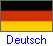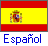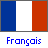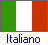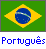1728 Software Systems'
Compound Interest Calculator
Calculates Annual, Semi-Annual, Quarterly, Monthly, Weekly, Daily and Continuously Compounded Interest.
Revised February, 2017 - To use the OLD Compound Interest Calculator click here.

Total = Principal × ( 1 + Rate )years

To see all four compound interest formulas, (semi-annual, quarterly, monthly and daily compounding) click here.
To see all four continuously compound interest formulas, (solved for total, principal, years and rate) click here.
 The above equation computes the total money you have after investing one lump sum (the principal) at a specified rate for a specified number of years. This calculator can solve for any one of these 4 numbers. Simply click on the button you don't know, input the other 3 numbers, then click on the method of compounding to get your answer. For example, you've just deposited \$5,000 (principal) at 9% interest compounded annually (rate) and now you are waiting for it to "grow" into \$10,000 (total). How long (years) will it take?
 Since you don't know the years, click on that button. Now enter the 3 numbers that you do know. Click on compounded "Annually" and your answer will be 8.0432 years. When inputting, do NOT use the dollar sign (\$), commas or the per cent sign (%). (For an interesting example of compound interest, scroll just beyond the calculator).
Do you want to solve for:

or or or
>>>>>
>>>>>
>>>>>
Compounded:

Effective Annual Rate Decimal Places
If you want to increase or decrease the accuracy of the Effective Annual Rate,
change the number in this box, then reclick the compounding method.

 Let us suppose you are 20 years old and wish to retire at age 65. What amount of money would you have to deposit at a 10 per cent rate compounded annually for this one deposit to make you a millionaire? We want to solve for principal so click on that button, then input: TOTAL = 1,000,000 YEARS =45 RATE = 10 per cent. Then click on compounded annually.
 Our answer shows that one deposit of \$13,719.21 at 10 per cent annual interest at age 20, allows you to be a millionaire at age 65. This shows you the incredible earning power of compound interest. It gives you something to think about doesn't it?